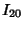## Deltahedron

A semiregular Polyhedron whose faces are all Equilateral Triangles. There are an infinite number of deltahedra, but only eight convex ones (Freudenthal and van der Waerden 1947). They have 4, 6, 8, 10, 12, 14, 16, and 20 faces. These are summarized in the table below, and illustrated in the following figures.Name 4 Tetrahedron 6 Triangular Dipyramid 8 Octahedron 10 Pentagonal Dipyramid 12 Snub Disphenoid 14 Triaugmented Triangular Prism 16 Gyroelongated Square Dipyramid 20 Icosahedron

The Stella Octangula is a concave deltahedron with 24 sides:

Another with 60 faces is a ``caved in'' Dodecahedron which is Icosahedron Stellation.

Cundy (1952) identifies 17 concave deltahedra with two kinds of Vertices.

See also Gyroelongated Square Dipyramid, Icosahedron, Octahedron, Pentagonal Dipyramid, Snub Disphenoid Tetrahedron, Triangular Dipyramid, Triaugmented Triangular Prism

References

Cundy, H. M. ``Deltahedra.'' Math. Gaz. 36, 263-266, 1952.

Freudenthal, H. and van der Waerden, B. L. ``On an Assertion of Euclid.'' Simon Stevin 25, 115-121, 1947.

Gardner, M. Fractal Music, Hypercards, and More: Mathematical Recreations from Scientific American Magazine. New York: W. H. Freeman, pp. 40, 53, and 58-60, 1992.

Pugh, A. Polyhedra: A Visual Approach. Berkeley, CA: University of California Press, pp. 35-36, 1976.